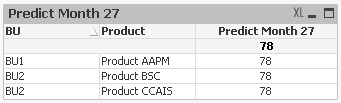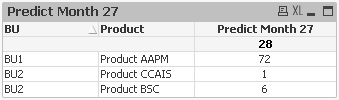# QlikView Layout & Visualizations

Discussion Board for collaboration on QlikView Layout & Visualizations.

HighlightedNot applicable

## Linear regression in straight table

Hi community. I am trying to predict the number of defects in future months based on linear regression of data from prior months. I am trying to do this in a straight table which shows multiple products that live in different business units (BU). My problem is that unless I filter on a single product the formula is returning the same value for all products. I would guess it has something to do with the aggr or total functions in my expression or calculated dimension, but can’t seem to figure out the problem. Can someone please look at the code below or the attached sample QVW and let me know what I’m doing wrong?

Calculated Dimension for Product (used to exclude products that don’t have data relevant to my table):

=aggr(only({<[Product]= P([Product]),[Data Source]= {'Second'},[Data Metric]= {'KPI Two'}>}[Product]),[Product])

Expression:

=linest_m(total aggr(if( [Month Num]>=14 and [Month Num]<=25 ,count({<[Include Flag]={1}>} distinct [Case Number])),[Month Num]),[Month Num])*27
+
linest_b(total aggr(if( [Month Num]>=14 and [Month Num]<=25 ,count({<[Include Flag]={1}>} distinct [Case Number])),[Month Num]),[Month Num])

Output:Thanks so much.

1 Solution

Accepted SolutionsNot applicable

## Re: Linear regression in straight table

I believe I found the answer in the following thread ... Multi-dimensional regression analysis

Here is my revised expression:

=linest_m(total <BU,Product> aggr(if( [Month Num]>=14 and [Month Num]<=25,count({<[Include Flag]={1}>} distinct [Case Number])),BU,Product,[Month Num]),[Month Num])*27
+
linest_b(total <BU,Product> aggr(if( [Month Num]>=14 and [Month Num]<=25,count({<[Include Flag]={1}>} distinct [Case Number])),BU,Product,[Month Num]),[Month Num])

Here are the revised results:Not applicable

## Re: Linear regression in straight table

I believe I found the answer in the following thread ... Multi-dimensional regression analysis

Here is my revised expression:

=linest_m(total <BU,Product> aggr(if( [Month Num]>=14 and [Month Num]<=25,count({<[Include Flag]={1}>} distinct [Case Number])),BU,Product,[Month Num]),[Month Num])*27
+
linest_b(total <BU,Product> aggr(if( [Month Num]>=14 and [Month Num]<=25,count({<[Include Flag]={1}>} distinct [Case Number])),BU,Product,[Month Num]),[Month Num])

Here are the revised results: